Courses

# RD Sharma Solutions Ex-6.2, Factorization Of Polynomials, Class 9, Maths Class 9 Notes | EduRev

## Class 9 : RD Sharma Solutions Ex-6.2, Factorization Of Polynomials, Class 9, Maths Class 9 Notes | EduRev

The document RD Sharma Solutions Ex-6.2, Factorization Of Polynomials, Class 9, Maths Class 9 Notes | EduRev is a part of the Class 9 Course RD Sharma Solutions for Class 9 Mathematics.
All you need of Class 9 at this link: Class 9

Q1. If f(x) = 2x3–13x2+17x+12 , Find

1. f(2)
2. f(-3)
3. f(0)

Sol :

The given polynomial is f(x) = 2x3–13x2+17x+12

1. f(2)

we need to substitute the ‘2‘ in f(x)

f(2) = 2(2)3–13(2)2+17(2)+12

= (2 * 8) – (13 * 4) + (17 * 2) + 12

= 16 – 52 + 34 + 12

= 10

therefore f(2) = 10

2. f(-3)

we need to substitute the ‘ (-3) ‘ in f(x)

f(-3) =2(−3)3–13(−3)2+17(−3)+12

= (2 * -27) – ( 13 * 9) – ( 17 * 3) + 12

= -54 – 117 – 51 + 12

= -210

therefore f(-3) = -210

3. f(0)

we need to substitute the ‘(0)‘ in f(x)

f(0) = 2(0)3–13(0)2+17(0)+12

= ( 2 * 0) – ( 13 * 0) + (17 * 0) + 12

= 0 – 0 + 0 + 12

= 12

therefore f(0) = 12

Q2. Verify whether the indicated numbers are zeros of the polynomial corresponding to them in the following cases :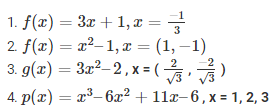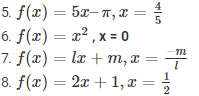Sol :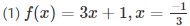we know that ,

f(x) = 3x + 1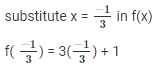= -1 + 1

= 0

Since, the result is 0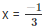is the root of 3x + 1

(2) f(x) = x2–1,x = (1,−1)

we know that,

f(x) = x2 – 1

Given that x = (1 , -1)

substitute x = 1 in f(x)

f(1) = 12 – 1

= 1 – 1

= 0

Now , substitute x = (-1) in f(x)

f(-1) = (−1)2 – 1

= 1 – 1

= 0

Since , the results when x = (1 , -1) are 0 they are the roots of the polynomial f(x) = x2 – 1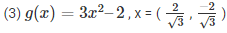Sol :

We know that

g(x) = 3x2–2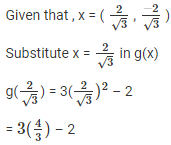= 4 – 2

= 2 ≠ 0
Now, Substitute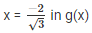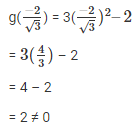Since, the results when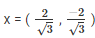are not 0, they are roots of 3x2–2

(4) p(x) = x3–6x2+11x–6 , x = 1, 2, 3

Sol :

We know that ,

p(x) = x3–6x2+11x–6

given that the values of x are 1, 2 , 3

substitute x = 1 in p(x)

p(1) = 13–6(1)2+11(1)–6

= 1 – (6 * 1) + 11 – 6

= 1 – 6 + 11 – 6

= 0

Now, substitute x = 2 in p(x)

P(2) =23–6(2)2+11(2)–6

= (2 * 3) – ( 6 * 4) + ( 11 * 2) – 6

= 8 – 24 – 22 – 6

= 0

Now, substitute x = 3 in p(x)

P(3) = 33–6(3)2+11(3)–6

= ( 3 * 3) – (6 * 9) + (11 * 3) – 6

= 27 – 54 + 33 – 6

= 0

Since , the result is 0 for x = 1, 2, 3 these are the roots of x3–6x2+11x–6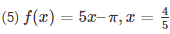we know that ,

f(x) = 5x–π

Given that , x = 4/5

Substitute the value of x in f(x)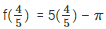= 4 – π

≠ 0

Since , the result is not equal to zero ,  x = 4/5 is not the root of the polynomial 5x – π

(6) f(x) = x2 , x = 0

Sol :

we know that , f(x) = x2

Given that value of x is ‘ 0 ’

Substitute the value of x in f(x)

f(0) = 02

= 0

Since, the result is zero , x = 0 is the root of x2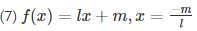Sol :

We know that,

f(x) = lx + m

Given , that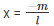Substitute the value of x in f(x)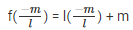= -m + m

= 0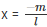is the root of lx + m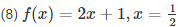Sol :

We know that ,

f(x) = 2x + 1

Given that x = 1/2

Substitute the value of x and f(x)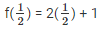= 1 + 1

= 2 ≠ 0

Since , the result is not equal to zero

x = 1/2 is the root of 2x + 1

Q3. If x = 2 is a root of the polynomial f(x) = 2x2–3x+7a, Find the value of a

Sol :

We know that , f(x) = 2x2–3x+7a

Given that x = 2 is the root of f(x)

Substitute the value of x in f(x)

f(2) = 2(2)2–3(2)+7a

= (2 * 4) – 6 + 7a

= 8 – 6 + 7a

= 7a + 2

Now, equate 7a + 2 to zero

⇒ 7a + 2 = 0

⇒ 7a = -2

⇒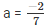The value ofQ4. If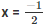is zero of the polynomial p(x) = 8x3–ax2–x+2 , Find the value of a

Sol :

We know that , p(x) = 8x3–ax2–x+2

Given that the value of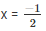Substitute the value of x in f(x)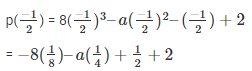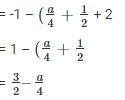To , find the value of a , equate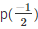to zero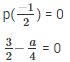On taking L.C.M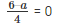⇒ 6 – a = 0

⇒ a = 6

Q5. If x = 0 and x = -1 are the roots of the polynomial f(x) = 2x3–3x2+ax+b , Find the of a and b.

Sol :

We know that ,  f(x) = 2x3–3x2+ax+b

Given , the values of x are 0 and -1

Substitute x = 0 in f(x)

f(0) = 2(0)3–3(0)2+a(0)+b

= 0 – 0 + 0 + b

= b     ——— 1

Substitute x = (-1) in f(x)

f(-1) = 2(−1)3–3(−1)2+a(−1)+b

= -2 – 3 – a + b

= -5 – a + b    ———— 2

We need to equate equations 1 and 2 to zero

b = 0 and -5 – a + b = 0

since, the value of b is zero

substitute  b = 0 in equation 2

⇒ -5 – a = -b

⇒ -5 – a = 0

a = -5

the values of a and b are -5 and 0 respectively

Q6. Find the integral roots of the polynomial f(x) = x3+6x2+11x+6

Sol :

Given , that f(x) = x3+6x2+11x+6

Clearly we can say that, the polynomial f(x) with an integer coefficient and the highest degree term coefficient which is known as leading factor is 1.

So, the roots of f(x) are limited to integer factor of 6, they are

±1, ±2, ±3, ±6

Let x = -1

f(-1) = (−1)3+6(−1)2+11(−1)+6

= -1 + 6 -11 + 6

= 0

Let x = -2

f(-2) = (−2)3+6(−2)2+11(−2)+6

= -8 – (6 * 4) – 22 + 6

= -8 + 24 – 22 + 6

= 0

Let x = -3

f(-3) = (−3)3+6(−3)2+11(−3)+6

= -27 – (6 * 9) – 33 + 6

= -27 + 54 – 33 + 6

= 0

But from all the given factors only -1 , -2 , -3 gives the result as zero .

So, the integral multiples of x3+6x2+11x+6 are -1 , -2 , -3

Q7. Find the rational roots of the polynomial f(x) = 2x3+x2–7x–6

Sol :

Given that f(x) = 2x3+x2–7x–6

f(x) is a cubic polynomial with an integer coefficient . If the rational root in the form of p/q , the values of p are limited to factors of 6 which are ±1, ±2, ±3, ±6

and the values of q are limited to the highest degree coefficient i.e 2 which are ±1, ±2

here, the possible rational roots are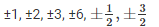Let , x = -1

f(-1) = 2(−1)3+(−1)2–7(−1)–6

= -2 + 1 +7 – 6

= -8 + 8

= 0

Let , x = 2

f(-2) = 2(2)3+(2)2–7(2)–6

= ( 2 * 8) + 4 -14 – 6

= 16 + 4 -14 – 6

= 20 – 20

= 0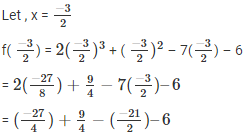But from all the  factors only -1 , 2 and −3/2 gives the result as zero

So, the rational roots of 2x3+x2–7x–6 are -1, 2 and −3/2

Offer running on EduRev: Apply code STAYHOME200 to get INR 200 off on our premium plan EduRev Infinity!

91 docs

,

,

,

,

,

,

,

,

,

,

,

,

,

,

,

,

,

,

,

,

,

,

,

,

,

,

,

,

,

,

;